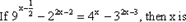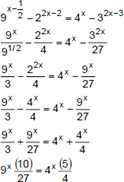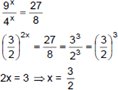# CAT 2017 – Slot 2 – Quantitative Ability – If the product of three consecutive positive integers is 15600

Q. 1: If the product of three consecutive positive integers is 15600 then the sum of the squares of these integers is
A) 1777
B) 1785
C) 1875
D) 1877

Explanation:- Given, (n – 1) (n) (n + 1) = 15600

As 15600 has 2 zeroes in it, one of n – 1, n or n + 1 should be a multiple of 25.  Dividing 15600 by 25, we get 624, but 624 = 24*26 so, the numbers are 24, 25 and 26

24^2 + 25^2 + 26^2 = 1877

##### Q. 2: If x is a real number such that log(base 3)5 = log(base 5)(2 + x), then which of the following is true?A) 0 < x < 3B) 23 < x < 30C) x > 30D) 3 < x < 23

Log(base 3)5 lies between 1 and 2 because Log(base 3)3 = 1 and Log(base 3)9 = 2

1 < Log(base 3)5 < 2

So, log(base 5)(2+x) should also lie between 1 and 2

1 < log(base 5)(2+x) < 2

5^1 < 2+x < 5^2

5 < 2+x < 25

3 < x < 23

Option D is the right answer.

Q. 3: Let f(x) = x^2 and g(x) = 2^x, for all real x. Then the value of f(f(g(x)) + g(f(x))) at x = 1 is
A) 16
B) 18
C) 36
D) 40

f(g(x)) = 2^(2x)

g(f(x)) = 2^((x)^2)

f(f(g(x)) + g(f(x)) = (2^(2x) + 2^(x^2))^2

at x = 1, we get 36

Option (C)

Q. 4: The minimum possible value of the sum of the squares of the roots of the equation x^2 + (a + 3)x – (a + 5) = 0 is
A) 1
B) 2
C) 3
D) 4

b and c can be the roots of the given equation.

We have to find, b^2 + c^2 = (b+c)^2 – 2bc

b+c = -(a+3) and bc = -(a+5)

b^2 + c^2 = (a+3)^2 + 2(a+5) = a^2 + 8a + 19

Min value of a quadratic equation = -Discriminant (D)/4*First term

D = b^2 – 4ac = 64 – 76 = -12

Min value = 12/4 = 3

Option (C)

Q. 5:A) 3/2
B) 2/5
C) 3/4
D) 4/9Alternately, we could’ve substituted the value of x from the options and it would’ve taken much less time.

##### Checkout Other Questions of CAT 2017 Slot 2 Paper:

Verbal Ability :              |   Q.01- Q.06  |  Q.07- Q.12  |  Q.13- Q.18  |  Q.19- Q.21  |  Q.22- Q.24  |  Q.25- Q.29  |  Q.30 – Q.34  |

Logical Reasoning :    |   Q.29 – Q.32  |

Quantitative Aptitude: |

### Free Material Area